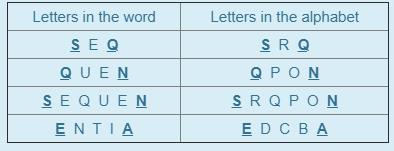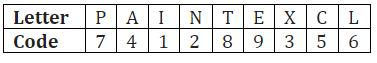Courses

# Coding - Decoding MCQ 1

## 20 Questions MCQ Test General Intelligence and Reasoning for SSC CHSL | Coding - Decoding MCQ 1

Description
This mock test of Coding - Decoding MCQ 1 for LR helps you for every LR entrance exam. This contains 20 Multiple Choice Questions for LR Coding - Decoding MCQ 1 (mcq) to study with solutions a complete question bank. The solved questions answers in this Coding - Decoding MCQ 1 quiz give you a good mix of easy questions and tough questions. LR students definitely take this Coding - Decoding MCQ 1 exercise for a better result in the exam. You can find other Coding - Decoding MCQ 1 extra questions, long questions & short questions for LR on EduRev as well by searching above.
QUESTION: 1

### How many pairs of letters are there in the word 'SEQUENTIAL' which have as many letters between them as are in the alphabet?

Solution:QUESTION: 2

### If PINK is written as MLKN, how will ROSE be written?

Solution:

P-3 = M  ,  I+3 = L  ,  N-3 = K  ,  K+3 = N. (PINK = MLKN )

IN THAT WAY ROSE = ORPH

QUESTION: 3

### If SELDOM is written as MFT LNC, how will PRINCE be written?

Solution:

S E L D O M - M F T L N C

First concentrate on first 3 letters that are SEL, now see the first 3 letters of the code that are MFT.

S. E. L & M. F. T

Now, if we see the next letter of S that is T and of L that is M and of E that is F.

In the same way when we take last 3 letters from the word D. O. M & L. N. C Following the same pattern from above but there is a little change. We need to write preceding letters like for D it is C and for M it is L and for O it is N.

QUESTION: 4

If GREAT is written as BVBCS, how will UNTIL be written?

Solution:

The last letter of great T comes after last letter of BVBCS
S = T - 1
C = A + 2
B = E - 3
V = R + 4
B = G - 5

similarly for UNTIL.

U - 5 = P

N + 4 = R

T - 3 = Q

I + 2 = K

L - 1 = K

QUESTION: 5

If OPPOSITE is written as NOQPTJSD, how will STRAIGHT be written?

Solution:

The mentioned rule is followed in word "OPPOSITE" the same will be followed in word "STRAIGHT".-1 -1 +1 +1 +1 +1 -1 -1

QUESTION: 6

If STUDENT is written as RRTBDLS, how will OFFICER be written?

Solution:

Answer is B because if we see the first word example

S    -1 = R

T    -2 = R

U    -1 = T

D    -2 = B

E    -1 = D

N    -2 = L

T    -1 = S

in the same logic of alternatively moving 1 and 2 letters backward we get OFFICER and NDEGBCQ

QUESTION: 7

In a certain code LAWN is written as JCUP. How will SLIT be coded in that code?

Solution:

Its all in the manner of -2
J=L-2
C=A-2
U=W-2
P=N-2
and same for SLIT.

QUESTION: 8

If TOUR is written in a certain code as 1234, CLEAR as 56784 and SPARE as 90847, what will be the 5th digit for SCULPTURE in the same code?

Solution:

By, comparing letters and digit, we get
T = 1
O = 2
U = 3
R = 4
C = 5
L = 6
E = 7
A = 8
S = 9 and
P = 0
As the 5th letter is SCULPTURE is P and '0' is used for P, therefore, 5th digit is the required code which is '0'.

QUESTION: 9

If POSTPONE is written as OPTSOPEN, how will RAJASTAN be written?

Solution:

Every letter is interchanged by it's subsequent second letter

QUESTION: 10

If BANGALORE is written as CZOFBKPQF, how will CALCUTTA be written?

Solution:

This letter is coded on the basis of +1 and -1 concept.. B + 1 = C, A - 1 = Z, N + 1 = O, G - 1 = F and so on

QUESTION: 11

If EXPLAINING is written as PXEALNIGNI, how will PRODUCTION be written?

Solution:

In the code, first three letters are reversed, then next two letters, then again next two letters and finally the last three letters are revesed in order.Using the same correct option is D.

QUESTION: 12

If TEACHING is written as CHEATING, how will BANKINGS be written?

Solution:
QUESTION: 13

In a certain code FIRE is coded as DGPC. What will be the last letter of the coded word for SHOT.

Solution:

D is used for F.
G is used for I.
P is used for R.
and
C is used for E. Thus, it is clear that each letter of the word FIRE stands for each corresponding letter of the coded word DGPC two places ahead. By applying the same principle, the letters QFMR will stand for SHOT. Hence, the last letter of the coded word is R.

QUESTION: 14

If AWAKE is written as ZVZJD, how will FRIEND be written?

Solution:

A -1 = Z

W-1 = V

A -1 = Z

K -1 = J

E -1 = D

similarly going one letter back FRIEND would be EQHDMC

QUESTION: 15

If in a code language PAINT is written as 74128 and EXCEL as 93596, then how ACCEPT will be written in that language?

Solution:The code for ACCEPT is 455978.

QUESTION: 16

If LOGIC is written as BHFNK, how will CLERK be written?

Solution:

When write the letters of the given word in reverse order and find its previous letters we get the answer.that is reverse of "LOGIC" is "CIGOL" Now write the previous letters of the word "CIGOL" as "BHFNK".

similarly  "CLERK" is written as "KRELC" and answer is "JQDKB".

QUESTION: 17

If LMNO is written as GHIJ, how will STUV be written?

Solution:

Subtract 5 from each letter
It goes in like...
L-5= G
M-5= H
So on.
And hence answer will be 'NOPQ'

QUESTION: 18

If AVOPDE is written as ATONCE, how will DONE AT GATV AT ZOU DAP be written?

Solution:

Here you can see the vowels are not changing ,only consonants are changing and they are also changing in the order of -2,-2,-1 so you get A

QUESTION: 19

If PREMONITION is written as 68530492904, how will MONITOR be written?

Solution:

Letters of the word PREMONITION are coded as follows:

Letters :  P    R    E    M    O    N    I    T    I    O    N

Code:        6    8    5    3    0    4    9    2    9    0    4

See the code below Is and Ns. Codes for I and N are 9 and 4 respectively. This indicates that the digits below each letters represent its code. Therefore, code for the word MONITOR is 3049208

QUESTION: 20

If REQUEST is written as S2R52TU, how will ACID be written?

Solution:

Vowels A, E, I, O, U are coded as 1, 2, 3, 4, 5 respectively. Each of the consonants in the word is moved one step forward to give corresponding letter of the code.
So ACID = 1D3E